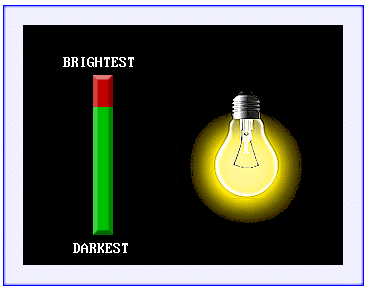# Touch Slider

This example illustrates how to implement the PROGRESS object as a touch slider. It utilizes the Standard CAN Create C Function int Touch.Read(int *X, int *Y)which reads the current coordinates being pressed.

Download the attached file below and open the CANcreate project (TouchSlider.ccs). Remember to import the CANcreate Image Pack by accessing Tools>Open Image Library>Import Image Pack, open the (TouchSlider.cip) file, and refresh/reload the library.

Before running the simulator, it is essential to compile the C-script first by clicking Publish>Compile.
With the simulator on, the progress bar will appear green as the brightness is increased and it will show red as it is decreased (pictured below in Figure 1-1).
As described in the Progress Bars example, the object’s property (range) should be set to the expected range of the input. For the purpose of this example, a range of 20 is used (but larger ranges are supported).
The SPRITE object was used to create the dimming effect of the light bulb.Figure 1-1

This example uses the parallel C-scripting to determine if the touch object is being pressed. The plain text code script can be accessed by clicking View>Code Script.
Alternatively, a code editor can be used to produce an external script (as illustrated in Figure 1-2). The code script file should then be imported by clicking View>Design Properties>Browse while enabling ‘Auto import external script on compile’.

In the TouchSlider.c file shown below, the code checks to see if the touch object (TCH_SLIDER) is being pressed. If it is, the Touch.Read function is executed which reads the current X and Y coordinates. Next, the Y coordinate read from the function is offset by 210 and stored as the variable TCH_OFFSET. The TCH_OFFSET is then divided by 8 (since the progress object Y coordinates have a difference of 160 and the range was set to 20) thereby determining what percentage of the full(green)/empty(red) graphic to interpolate between. The remainder of the code checks to see if the PROG_VAL (progress object value) will fall into one of six different conditions. For example, when the progress value is 16, XPOS = 4; therefore the sprite sheet source rectangle will be of size (150,150) at the location (600,150).

```int Y, val, XPOS,YPOS;
int TCH_OFFSET, PROG_VAL;

void Init()
{
}

void Update()
{
if (TCH_SLIDER)
{
TCH_OFFSET = 210 - Y;
PROG_VAL = (TCH_OFFSET / 8);

if (PROG_VAL <=1)
{
XPOS = 0;
} else if (PROG_VAL > 1 && PROG_VAL <= 5)
{
XPOS = 1;
} else if (PROG_VAL > 5 && PROG_VAL <= 10)
{
XPOS = 2;
} else if (PROG_VAL > 10 && PROG_VAL <= 15)
{
XPOS = 3;
} else if (PROG_VAL > 15 && PROG_VAL < 20)
{
XPOS = 4;
} else if(PROG_VAL >= 20)
{
XPOS = 5;
}
}
}```